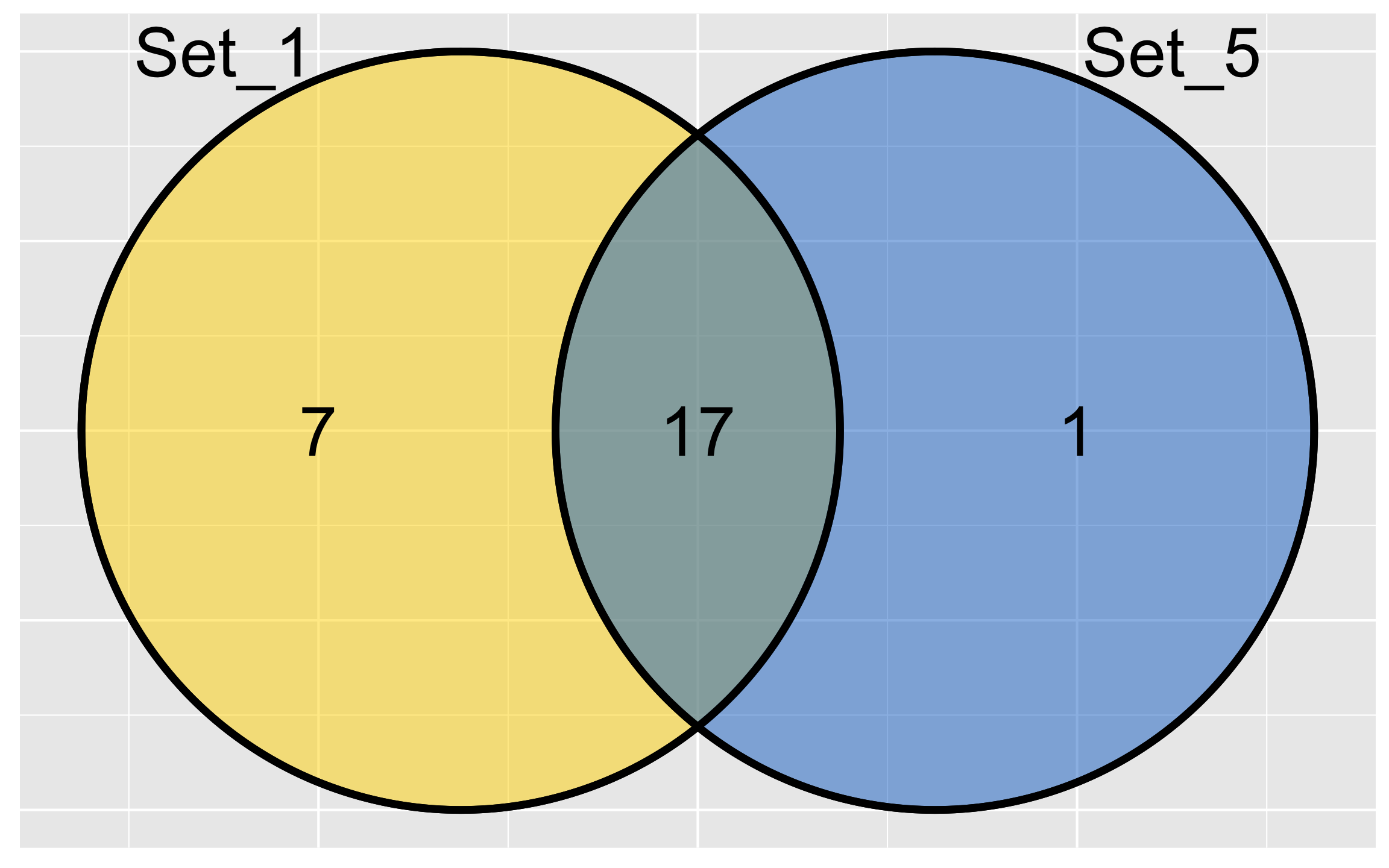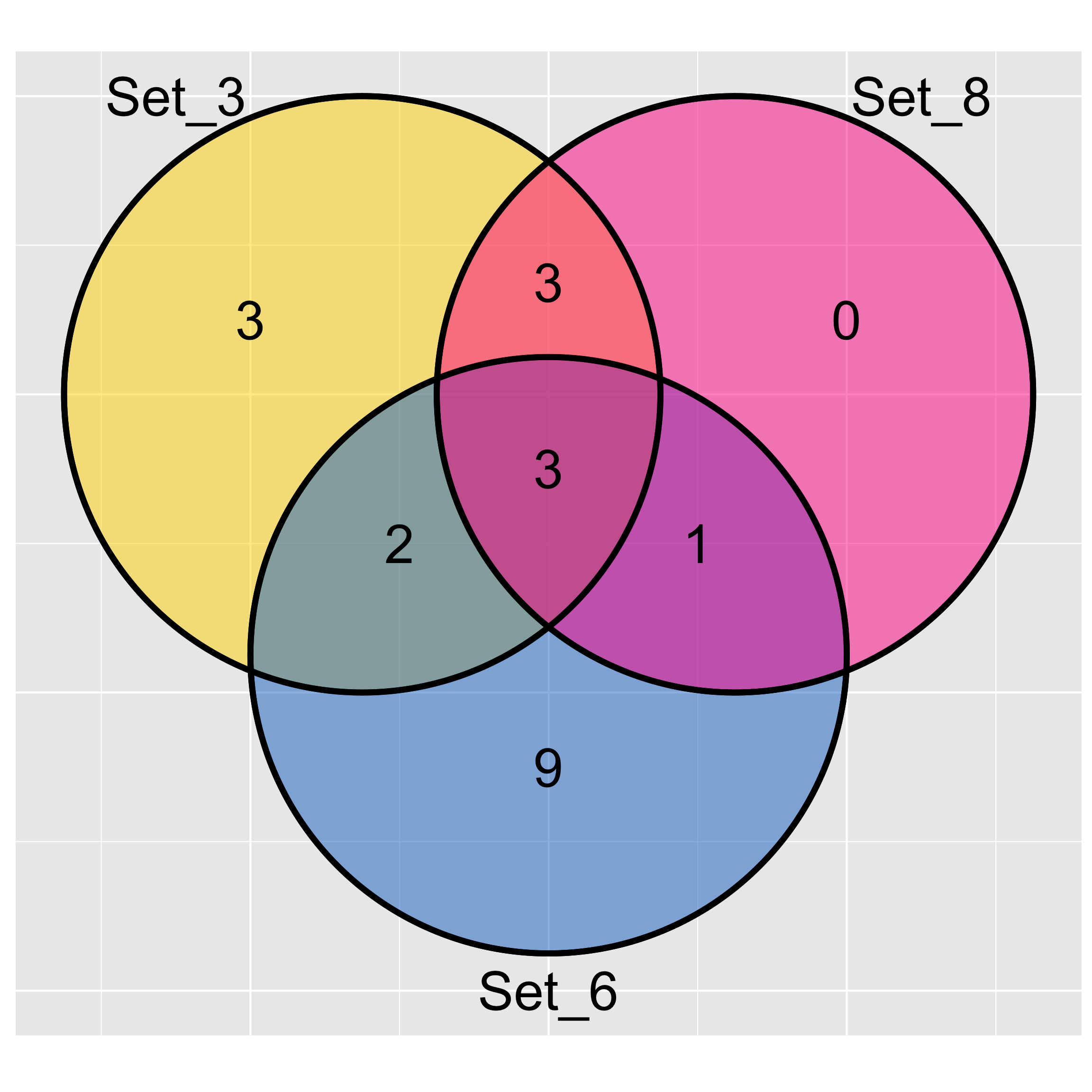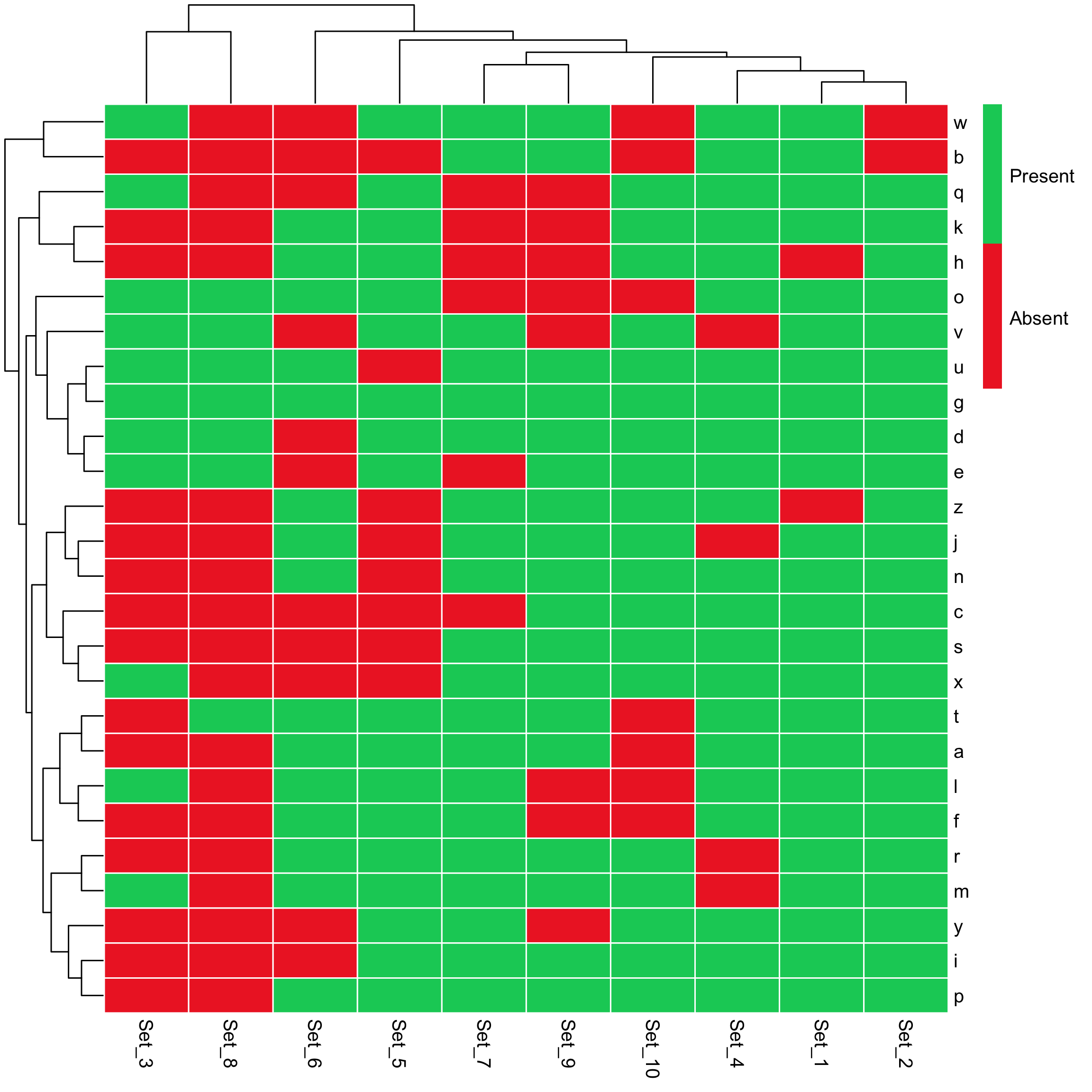RVenn: An R package for set operations on multiple sets

Introduction

This tutorial shows how to use RVenn, a package for dealing with multiple sets. The base R functions (intersect, union and setdiff) only work with two sets. %>% can be used from magrittr but, for many sets this can be tedious. reduce function from purrr package also provides a solution, which is the function that is used for set operations in this package. The functions overlap, unite and discern abstract away the details, so one can just construct the universe and choose the sets to operate by index or set name. Further, by using ggvenn Venn diagram can be drawn for 2-3 sets. As you can notice from the name of the function, ggvenn is based on ggplot2, so it is a neat way to show the relationship among a reduced number sets. For many sets, it is much better to use UpSet or setmap function provided within this package. Finally, by using enrichment_test function, the p-value of an overlap between two sets can be calculated. Here, the usage of all these functions will be shown.

Creating toy data

This chunk of code will create 10 sets with sizes ranging from 5 to 25.

library(purrr)
library(RVenn)
library(ggplot2)
set.seed(42)
toy = map(sample(5:25, replace = TRUE, size = 10),
function(x) sample(letters, size = x))
toy[1:3]  # First 3 of the sets.
#> []
#>   "l" "r" "w" "f" "k" "t" "u" "c" "i" "j" "o" "s" "n" "m" "a" "x" "d"
#>  "y" "q" "v" "e" "g" "b" "p"
#>
#> []
#>   "a" "u" "z" "e" "t" "m" "h" "i" "x" "q" "g" "o" "y" "s" "l" "p" "d"
#>  "j" "n" "f" "r" "v" "c" "k"
#>
#> []
#>   "g" "m" "q" "w" "x" "l" "v" "d" "e" "o" "u"

Venn Diagram

For two sets:

ggvenn(toy, slice = c(1, 5))For three sets:

ggvenn(toy, slice = c(3, 6, 8))Heatmap

setmap(toy)Without clustering

setmap(toy, element_clustering = FALSE, set_clustering = FALSE)